# CTF | 2021 春秋杯 Baby_steg WriteUp## 引言

2021年 春秋杯 网络安全联赛春季赛

https://www.ichunqiu.com/chunqiucup

## Baby_steg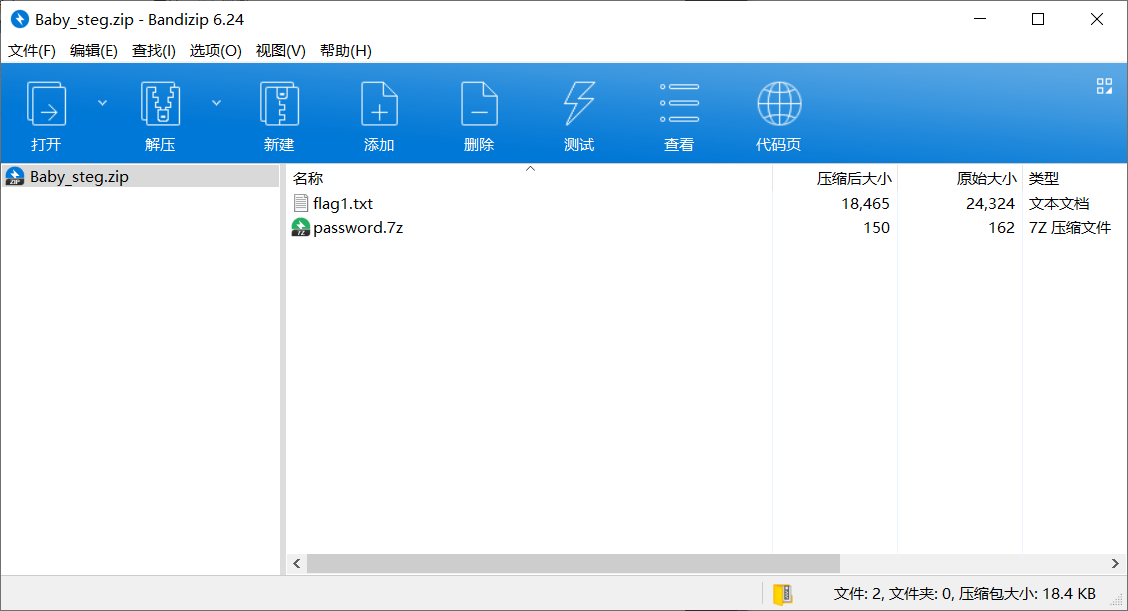``````##
\$1 *``````

``crark-7z.exe -l6 -g8 password.7z``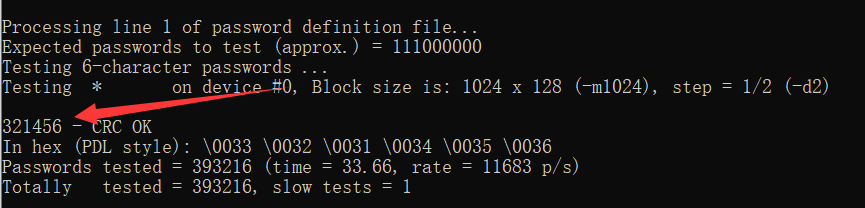``7324623c``

`flag1.txt` 这是什么玩意……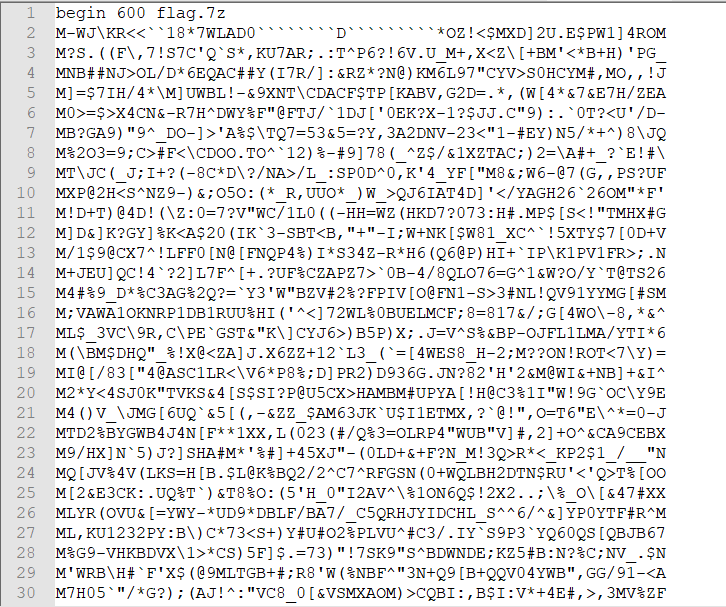``````import uu
s = uu.decode('flag1.txt')``````

``uu.encode(input_file, output_file)``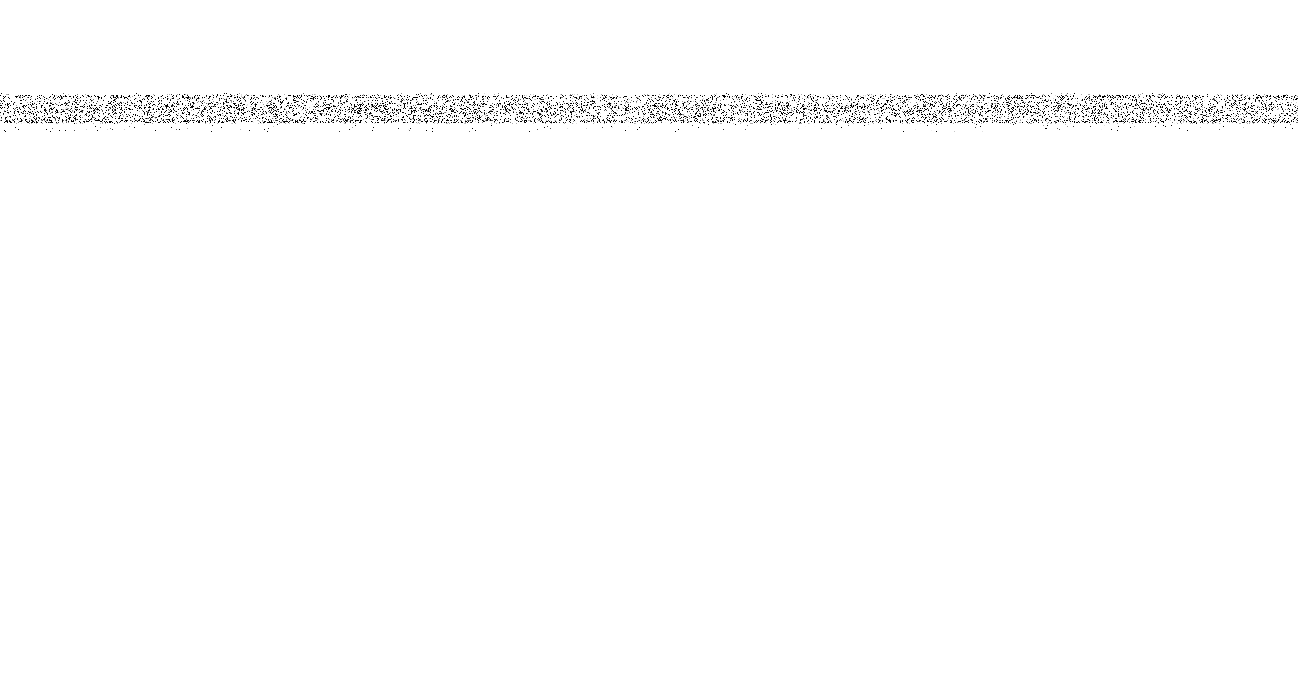``````import numpy as np
import cv2
import sys
import random

def encode(image):
i = random.randint(520,540)
np.random.seed(i)
# image = 1298 * 695
to_hide_array = np.asarray(to_hide)

for i in range(to_hide_array.shape):
np.random.shuffle(to_hide_array[i])

gray = cv2.cvtColor(to_hide_array, cv2.COLOR_BGR2GRAY)
cv2.imwrite('challenge.png', gray)
print("encode!")

def main():
if len(sys.argv) != 2:
print('error!')
exit(1)
encode(sys.argv)

if __name__ == '__main__':
main()``````

``````import numpy as np
import cv2
import sys
import random

def decode(image):
# image = 1298 * 695
to_hide_array = np.asarray(to_hide)
shape = to_hide_array.shape

for i in range(520, 541):
print('====>', i)
np.random.seed(i)
l1 = []
for j in range(shape):
l2 = list(range(shape))
new_array = []
np.random.shuffle(l2)
for x in range(len(l2)):
new_array.append(to_hide_array[j][l2.index(x)])
l1.append(new_array)
l1_array = np.asarray(l1)
# gray = cv2.cvtColor(l1_array, cv2.COLOR_BGR2GRAY)
cv2.imwrite(f'decode{i}.png', l1_array)

def main():
decode('challenge.png')

if __name__ == '__main__':
main()``````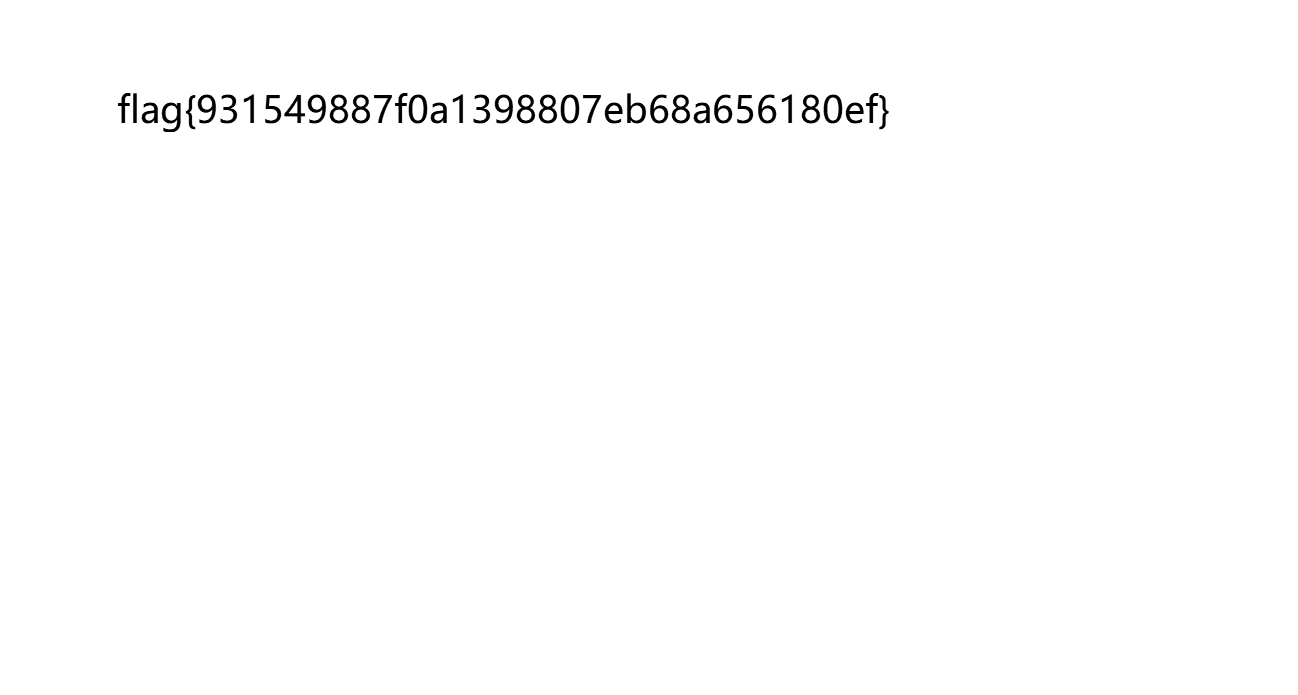## 小结

Baby_steg 这题还是堆了几个没玩过的知识点的，正好还把 7z 压缩包爆破的工具也找了一波，cRARk for 7-Zip 还是挺香的！

BTW，XCTF final 的题目太顶了，喵喵好菜玩不来，喵呜呜呜。

（看啥时候有机会再摸鱼来复现吧，喵喵🐱

（溜了溜了喵

上一篇CTF | 2021 DASCTF May WriteUp

2021-05-30
目录